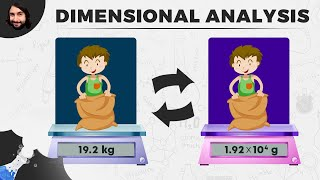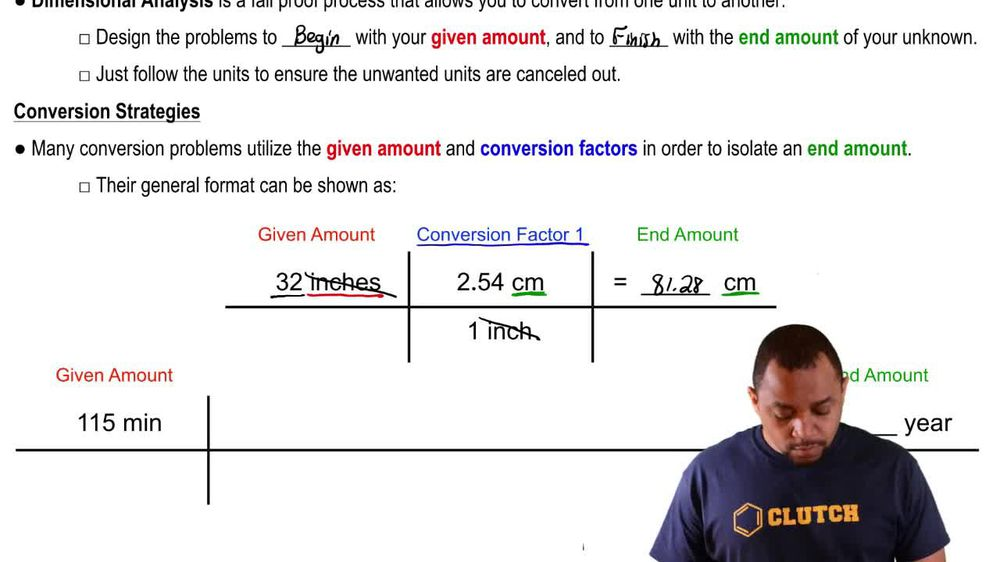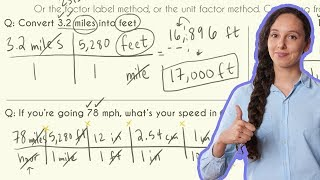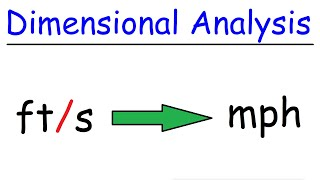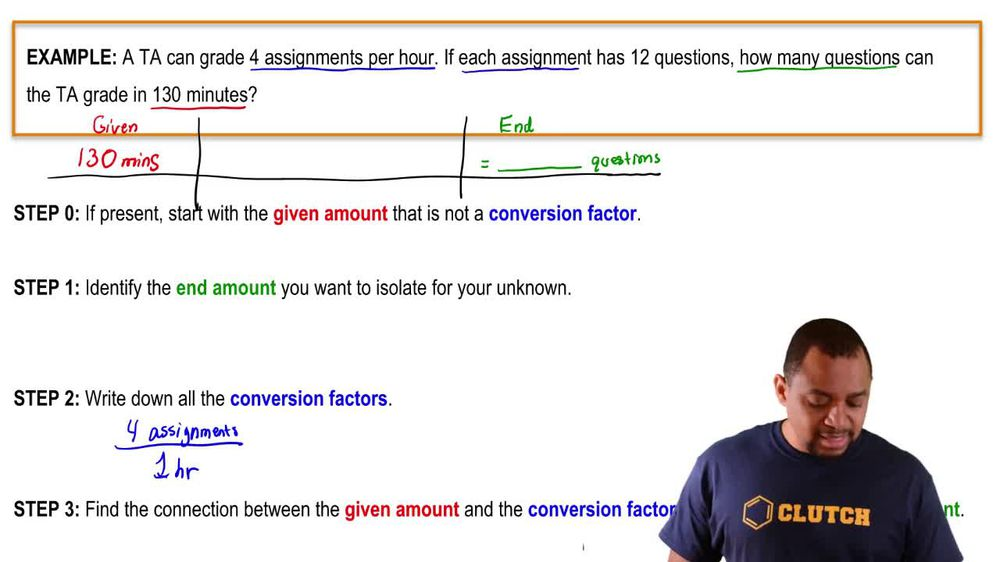Start typing, then use the up and down arrows to select an option from the list.
1. 1. Intro to General Chemistry2. Dimensional Analysis
Problem

# The volume of water used for crop irrigation is measured in acre-feet, where 1 acre-foot is the amount of water needed to cover 1 acre of land to a depth of 1 ft. (a) If there are 640 acres per square mile, how many cubic feet of water are in 1 acre-foot? (b) How many acre-feet are in Lake Erie (total volume = 116 mi^3)?

Relevant Solution2m
Play a video:
Hi everyone today, we have a question telling us that Earth has a radius of km around the equator, assuming the Earth as the perfect square, calculate the volume of Earth and cubic feet. So we're going to use the formula volume equals four thirds times pi times radius cubed. And we need to remember the conversion factors one km Equals 10 to the 3rd m and one m Equals 3.281 the cube. So now we need to convert our radius from km two ft. So we're going to start out with our 378 km And we're going to multiply that by 10 to the 3rd m over one kilometer. And we're going to multiply that by 3. ft per one m And that equals 2.09, 3 times 10 To the 7th ft. And now we're going to plug that into our volume equation. So our volume is going to equal 4/ pie Times our radius. We just calculated so 2.09, 3 Times 10 to the 7th. See, and this is cube And that equals three 841 times 10 To the 22nd feet cubed. And that is our final answer. Thank you for watching. Bye